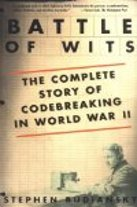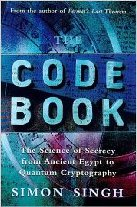## Introduction §

The ADFGX cipher was a field cipher used by the German Army during World War I. It is closely related to the ADFGVX cipher. ADFGX is a fractionating transposition cipher which combined a modified Polybius square with a single columnar transposition. The cipher is named after the five possible letters used in the ciphertext: A, D, F, G and X. These letters were chosen deliberately because they sound very different from each other when transmitted via morse code. The intention was to reduce the possibility of operator error.

## The Algorithm §

The 'key' for a ADFGX cipher is a 'key square' and a key word. e.g.

```p h q g m
e a y n o
f d x k r
c v s z w
b u t i l
```

The key square is a 5 by 5 square containing all the letters except 'j'. The key word is any word e.g. GERMAN

There are a number of steps involved:

1. Build a table like the following with the key square. This is known as a polybius square.

```    A D F G X
A | p h q g m
D | e a y n o
F | f d x k r
G | c v s z w
X | b u t i l
```
2. Encode the plaintext using this matrix, to encode the laetter 'a', locate it in the matrix and read off the letter on the far left side on the same row, followed by the letter at the top in the same column. In this way each plaintext letter is replaced by two cipher text letters. E.g. 'attack' -> 'DD XF XF DD GA FG'. The ciphertext is now twice as long as the original plaintext. Note that so far, it is just a simple substitution cipher, and trivial to break.

3. Write the code word with the enciphered plaintext underneath e.g.

```G E R M A N
D D X F X F
D D G A F G
```
4. Perform a columnar transposition. Sort the code word alphabetically, moving the columns as you go. Note that the letter pairs that make up each letter get split apart during this step, this is called fractionating.

```A E G M N R
X D D F F X
F D D A G G
```
5. Read the final ciphertext off in columns.

-> XF DD DD FA FG XG

This cipher works in the same way as the ADFGVX cipher, see that page for a longer example.

## Javascript Implementation §

The keysquare in this example has been written on a single line. To convert to the square shape, simply write the first 5 characters on the first line, the second 5 characters on the second line etc.

Plaintext

keysquare =

keyword =

Ciphertext

## Other Implementations §

To encipher your own messages in python, you can use the pycipher module. To install it, use pip install pycipher. To encipher messages with the ADFGX cipher (or another cipher, see here for documentation):

```>>>from pycipher import ADFGX
>>>a.encipher('defend the east wall of the castle')
>>>a.decipher(_)
'DEFENDTHEEASTWALLOFTHECASTLE'
```

## Cryptanalysis §

This section is the same as the page on ADFGVX cipher since the ciphers are so similar.

The cipher presents several difficulties to the cryptanalyst. Ordinarily when breaking columnar transposition ciphers, anagramming is used to determine the key. Once the substitution step is introduced, however, this approach becomes impossible. The letter frequencies are also modified due to the fractionating nature of the cipher, which adds further difficulties.

The French cryptanalyst Painvin, who first broke the ADVGVX cipher, only managed to break it in specific circumstances. The exact circumstances he needed only occurred on days with very heavy traffic. His techniques are described in Friedman's book 'Military Cryptanalysis - Part IV', along with several other more general techniques.

Cryptanalysis of the ADFGVX cipher is also discussed in a paper called "Cryptanalysis of ADFGVX encipherment systems" in Proceedings of CRYPTO 84 on Advances in cryptology. Unfotunately this paper is not available to the public, however an extended abstract is available.

## ContentsBattle of Wits: The Complete Story of Codebreaking in World War II ASIN/ISBN: 978-0743217347 A good history of the breaking of Enigma Buy from Amazon.comThe Code Book: The Science of Secrecy from Ancient Egypt to Quantum Cryptography ASIN/ISBN: 978-1857028799 Simon Singh's 'The Code Book' is an excellent introduction to ciphers and codes Buy from Amazon.com